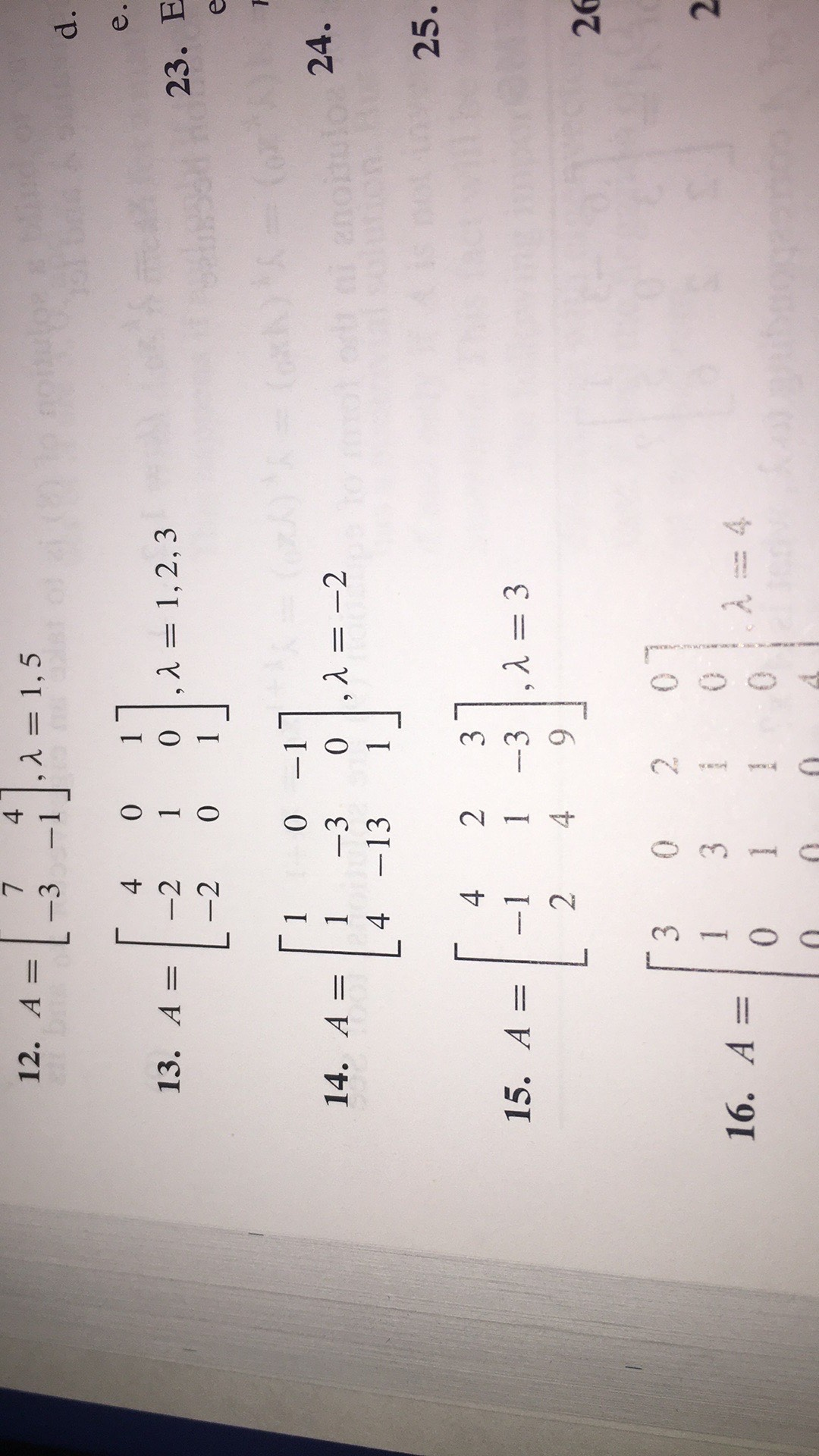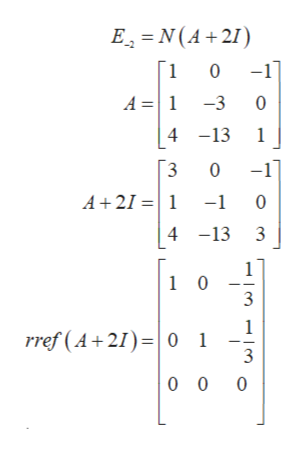# LA = 1,5-112. A =-3424C0II1, 2, 3e.13. A-2-20I23. Ee0 -1II-34 -1314. A =0,A = -224.2 DI25.2315. A =-1-3I426300T16. A=0A = 4T0U

Question
16 views

I am stuck on number 14. The directions say Find the basis for the eigenspace corresponding to each listed eigenvalue.help_outlineImage TranscriptioncloseL A = 1,5 -1 12. A = -3 42 4C 0 I I 1, 2, 3 e. 13. A -2 -2 0 I 23. E e 0 -1 I I -3 4 -13 14. A = 0 ,A = -2 24. 2 D I 25. 2 3 15. A = -1 -3 I 4 2 6 3 0 0 T 16. A= 0 A = 4 T 0 U fullscreen
check_circle

Step 1

By definition, the Eigen space corresponding to the Eigen value -2 is the null space of the matrix A+2I. Thus we have:help_outlineImage TranscriptioncloseEN(A 21) -11 1 0 A =1 -3 0 413 1 -1 3 A+211 -1 0 4 -13 3 1 1 0 3 1 rref (A 210 1 3 0 0 0 fullscreen
Step 2

Thus, the solution x of (A+2I) x = 0 satisfy x1 + x2 + 3x3 = 0 or x1 = - x2 - 3x3

Thus, the null space N(A+2I) consists of vectors.

Step 3

For any scaler x2 and x3.

Hence we have,

...

### Want to see the full answer?

See Solution

#### Want to see this answer and more?

Solutions are written by subject experts who are available 24/7. Questions are typically answered within 1 hour.*

See Solution
*Response times may vary by subject and question.
Tagged in

### Math